Electron. J. Diff. Equ., Vol. 2015 (2015), No. 67, pp. 1-16.

### Properties of Lyapunov exponents for quasiperodic cocycles with singularities Kai Tao

Abstract:
We consider the quasi-periodic cocycles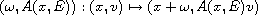withDiophantine. Let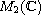be a normed space endowed with the matrix norm, whose elements are thematrices. Assume thatis jointly continuous, depends analytically on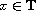and is Holder continuous in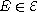, whereis a compact metric space and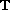is the torus. We prove that if two Lyapunov exponents are distinct at one point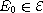, then these two Lyapunov exponents are Holder continuous at any E in a ball central at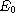. Moreover, we will give the expressions of the radius of this ball and the Holder exponents of the two Lyapunov exponents.

Submitted October 10, 2014. Published March 20, 2015.
Math Subject Classifications: 37C55, 37F10.
Key Words: Lyapunov exponent; quasiperodic cocycles; Holder exponent.

Show me the PDF file (280 KB), TEX file, and other files for this article.Kai Tao College of Sciences, Hohai University 1 Xikang Road Nanjing, Jiangsu 210098, China email: ktao@hhu.edu.cn, tao.nju@gmail.com